# Electronics and Communication Engineering - Exam Questions Papers

46.

The ratio of the diffusion coefficient in a semiconductor has the units

 A. V-1 B. em.V-1 C. V.cm-1 D. V.s

Explanation: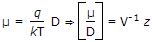.

47.

Consider the probability density f(x) = ae-b|x| where x is a random variable whose allowable values range from x = - ∞ to x = + ∞. P(1 ≤ x ≤ 2)

 A.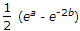B.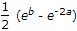C.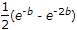D. (e-b - e-2b)

Explanation:

P(x) =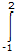ae-b|x|dx

to get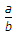apply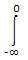aebxdx +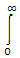ae-bxdx,

we get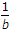=.

48.

The amplitude of a pair of composite sinusoidal signal y(n) = x1(n) + x2(n) with x1(n) = sin (5pn) x2(n) = 3 sin (5pn) is __________ .

 A. 2 B. 3 C. 4 D. 1

Explanation:

Amplitude =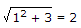.

49.

The amplifier circuit shown below uses a silicon transistor. The capacitors Cc and CE can be assumed to be short at signal frequency and the effect of output resistance r0 can be ignored. If CE is disconnected from the circuit, which one of the following statements is TRUE?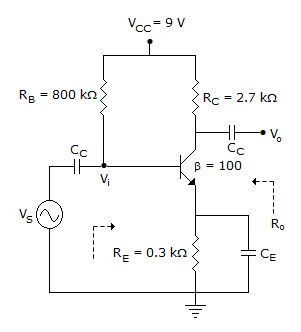A. The input resistance Ri increases and the magnitude of voltage gain Av decreases B. The input resistance Ri decreases and the magnitude of voltage gain Av decreases C. Both input resistance Ri and the magnitude of voltage gain Av decrease D. Both input resistance Ri and the magnitude of voltage gain Av increase

Explanation:

Given circuit after removing CE will behave as current-series feedback.

Overall voltage gain will decrease as feedback signal comes into picture and since it is current-series feedback, input impedance increases.

50.

A n-channel JFET has IDSS = 2 mA, Gate to source voltage VGS = - 2 V and trans-conductance is 0.5 mW then pinch-off voltage is __________ .

 A. - 2 V B. 2 V C. - 4 V D. 4 V

Explanation: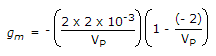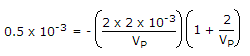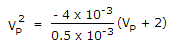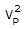= - 8VP - 16+ 8VP + 16 = 0

(VP + 4)2 = 0

VP = -4 V.